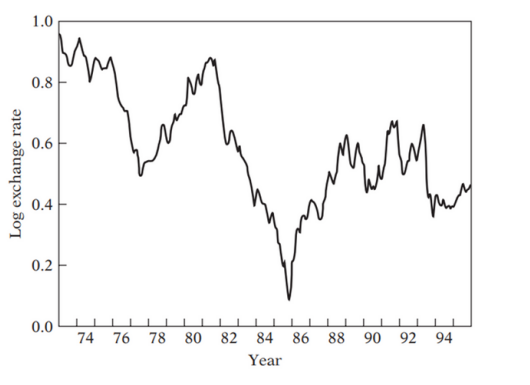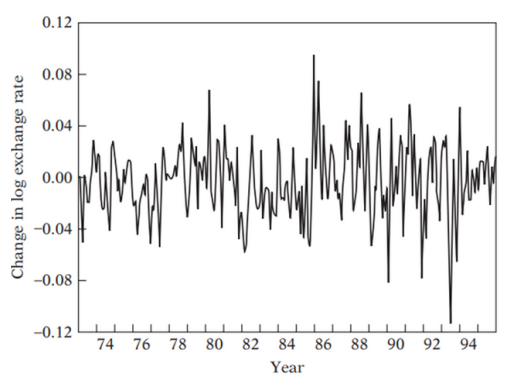# Autoregressive Conditional Heteroskedasticity (ARCH) Model

In econometrics, the autoregressive conditional heteroscedasticity (ARCH) model is a statistical model for time series data that describes the variance of the current error term or innovation as a function of the actual sizes of the previous time periods' error terms; often the variance is related to the squares of the previous innovations.

Financial time series such as stock, exchange rates, inflation rates, etc. often exhibit the phenomenon of volatility clustering that is periods in which their prices show wide swings for an extended time periods followed by periods in which there is relative calm.

If we see the Inflation rates, it is proved (based on macro econometric study) that inflation itself is not bad but the volatility in inflation rates is bad as it makes the financial planning difficult. The same is true for importers, exporters, exchange rates, stock prices and so on. Volatility in all these means huge losses or profit. In volatile market, it is difficult for a company to raise the capital in the capital market.

How do we model the financial time series that experiences such volatility? How do we model the inflation rates, stock prices, exchange rates? ARCH is one of the methods used to model this kind of financial time series.

Let’s understand the ARCH model by using U.S./U.K. exchange rates for the period 1973 to 1995.As you can see that there is a considerable up and down in the log exchange rates over a sample period of time. Let’s now plot the changes in the log exchange rates.

As you can observe, the relative change in the U.S./U.K. exchange rate show a period of wide wings for some time period and period of moderate swing in other time periods. This is the case of volatility clustering. In ARCH model, we model the volatility instead of actual data.

Y t = U.S./U.K. exchange rates
Y*t = Log of Yt
dY*t = Y*t - Y*t-1 (relative change in the exchange rates)
dÿ*t = Mean of dY*t
Xt = dY*t - dÿ*t (Mean adjusted relative change in exchange rates)

Now, we will use X2t to model the volatility using ARCH model. Its value will be high in periods when there are big changes in the prices of financial assets and low in periods when there are modest changes in the prices of financial assets.

X 2 t = β0 + β1 X 2 t-1 + β2 X 2 t-2 + …… + βp X 2 t-p + û

This ARCH model suggests that volatility (in the exchange rates) in the current period is related to volatility in the past p periods and some white noise error ( û ). Here, the lagged volatility can be determined by using information criteria like AIC, BIC, SC and usual t-test

Consider below regression model which contains Time Series data.

Yt = β0 + β1 X1 + β2 X2 + …… + βp Xp + û

We know the Normality of û . But variance of û at time t is new to us. This is what we need to model by using ARCH Model as follow:

Var(ût) = σ 2 t = β0 + β1 û 2 t - 1 + β2 û 2 t - 2 + …… + βp û 2 t – p

This model says the variance (volatility) at time t is a function of lagged squared error term. The output of this model would be volatility prediction. This is what we want to forecast in financial time series.

ARCH model Petameters are estimated by usual Maximum Likelihood method.

Difference between ARIMA and ARCH

An ARCH model is very similar to ARIMA model but the CH (conditional heteroskedasticity) component makes the ARCH model different. This CH component models the previous squared residuals (in our case, X 2 t or σ 2 t). The ARCH model output would be the volatility forecast and the ARIMA model output would be series forecast. If there is volatility say in Stock Price series, fitting ARIMA would miss the volatility which can be easily model by using ARCH.

Techinfoplace is a leading web solutions company that can provide you a complete range of products & solutions which help in improving your business by attracting more customers to raise your profits.

## Attended Events• Booth No.: E - 510
• EXHIBITION : 12 - 14 OCT 2017
• The Bombay Exhibition Centre at Goregaon East